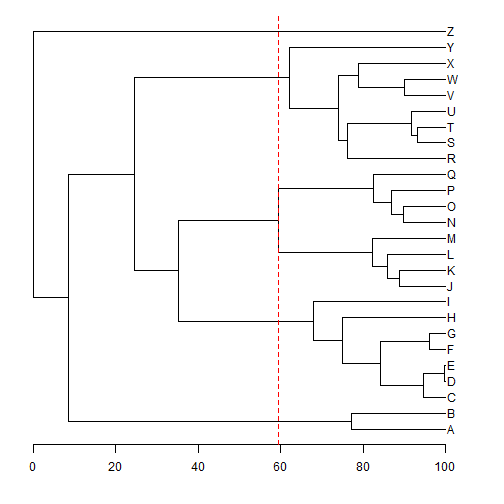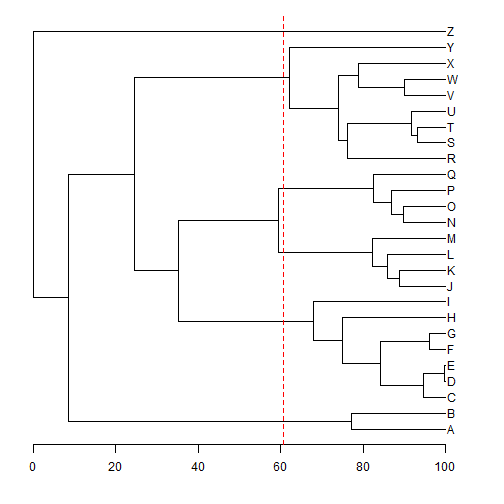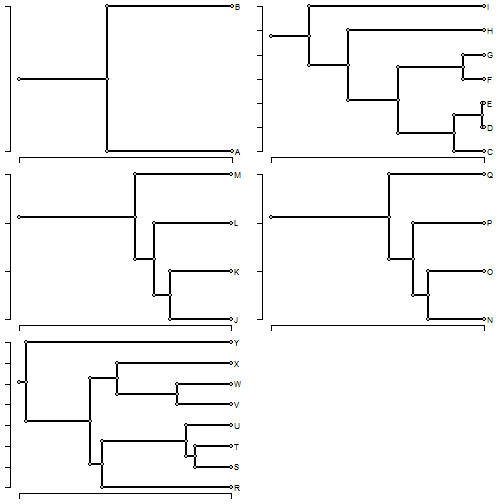## Saturday, April 9, 2016

### Drawing a line on (& slicing) a tree to create k subtrees

A phytools user recently asked the following:

I know this is very old post, but I am struggling to make a vertical line in my phylo plot for a given cut. The idea is drawing the line to show a cut of the dendrogram in k groups, rather than a given height.

OK, so we want to show a vertical line on a rooted tree at a point that demarcates k descendant subgroups? This is pretty easy, in fact. We can take advantage of the phytools function `ltt` as follows:

``````library(phytools)
tree
``````
``````##
## Phylogenetic tree with 26 tips and 25 internal nodes.
##
## Tip labels:
##  A, B, C, D, E, F, ...
##
## Rooted; includes branch lengths.
``````
``````obj<-ltt(tree,plot=FALSE)
obj
``````
``````## Object of class "ltt" containing:
##
## (1) A phylogenetic tree with 26 tips and 25 internal nodes.
##
## (2) Vectors containing the number of lineages (ltt) and branching times (times) on the tree.
##
## (3) A value for Pybus & Harvey's "gamma" statistic of 0.4726, p-value = 0.6365.
``````
``````## set k
k<-6
## find the height when there are k lineages
h<-obj\$times[which(obj\$ltt==k)]
## plot our tree
plotTree(tree,lwd=1,mar=c(4.1,1.1,1.1,1.1))
axis(1)
lines(rep(h,2),par()\$usr[3:4],col="red",lty="dashed")
``````The only trouble with this tactic is that because we have k subgroups at the event leading to the kth group, our line overlaps exactly the vertical line connecting the clades. Alternatively, we could split the difference between the 6th & 7th event and plot that line!

``````h<-mean(obj\$times[c(which(obj\$ltt==k),which(obj\$ltt==(k+1)))])
plotTree(tree,lwd=1,mar=c(4.1,1.1,1.1,1.1))
axis(1)
lines(rep(h,2),par()\$usr[3:4],col="red",lty="dashed")
``````Now we can clearly see that our vertical line cuts the tree at a point where there are k=6 descendant subtrees.

We can also physically slice the tree at this point if we are so inclined - using the phytools function `treeSlice`:

``````subtrees<-treeSlice(tree,h,trivial=TRUE)
print(subtrees,details=TRUE)
``````
``````## 6 phylogenetic trees
## tree 1 : 2 tips
## tree 2 : 7 tips
## tree 3 : 4 tips
## tree 4 : 4 tips
## tree 5 : 8 tips
## tree 6 : 1 tips
``````
``````par(mfrow=c(3,2))
lapply(subtrees,function(x) plotTree.singletons(rootedge.to.singleton(x)))
``````
``````## Error in if (attr(tree, "order") == "cladewise") cw <- tree else stop("tree must be in \"cladewise\" order."): argument is of length zero
``````Unfortunately, none of the plotting functions seem to like plotting trees with only one tip so we only get to see five of our six subtrees here. Nonetheless, you get the idea.